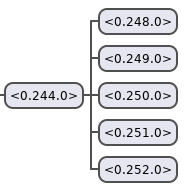Mostly code

# Advent of Code 2019: Day 7

09 Dec 2019More intcode computing! Without any modifications to the computer, and a quick search for an algorithm to make permutations, which, like, I just didn’t want to have to figure out, and I am ready to solve this.

``````  defp permutations([]), do: [[]]

defp permutations(list),
do: for(elem <- list, rest <- permutations(list -- [elem]), do: [elem | rest])
``````

Once my permutations are generated, all that’s left is to amp things up.

``````  defp amplify([p], prog, i),
do:
run(prog, [p, i])
|> (&List.first(&1.output)).()

defp amplify([p | r], prog, i) do
o =
run(prog, [p, i])
|> (&List.first(&1.output)).()

amplify(r, prog, o)
end

defp run(program, input, output \\ []) do
program
|> Intcode.new(input, output)
|> Intcode.run_all()
end
``````

Given a program and permutation of possible phase settings, run the 5 amplifiers, feeding the output of one into the input of the other. As there are `5! = 120` permutations that don’t affect each other, using `Task.async_stream/3` is a great reason to play with asynchronous processing. It takes my list of permutations and runs each one as a task, streaming the results to the next step in the pipeline. All that’s left is to find the highest frequency.

``````  def p1() do
input_stream()
|> Enum.at(0)
|> s1()
end

def s1(program) do
program
|> String.split(",")
|> Enum.map(&String.to_integer/1)
|> p(0..4, &amplify/3)
end

def p(program, phases, f) do
phases
|> Enum.to_list()
|> permutations()
fn frequencies ->
f.(frequencies, program, 0)
end,
timeout: :infinity
)
|> Enum.map(fn {:ok, i} -> i end)
|> Enum.max()
end
``````

I made the timeout here `:infinity` just in case these jobs took a lot longer than the default 5 seconds timeout (spoiler alert: they didn’t). That’s onein the bag!

## Puzzle 2

Feedback looping!? I can definitely connect inputs and outputs better. Hmm… Processes are a fundamental part of Erlang and Elixir… I know! I’ll wrap my computer in a process and `send/2` and `receive/1` messages between two computers! I need to add a new `input/2` that can handle if I’m supposed to receive input from somewhere else.

``````  defp input(%__MODULE__{program: prog, position: pos, input: :server} = computer, m) do
{:message, i} when is_integer(i) ->
%__MODULE__{computer | program: store(prog, shift(pos, 1), i, m), position: shift(pos, 2)}

{:output, o} when is_pid(o) or is_list(o) ->
input(%__MODULE__{computer | output: o}, m)

:halt ->
input(computer, m)

e ->
end
end
``````

My computer can now handle 3 different messages: `:message`, `:output`, and `:halt`. `:message` is used to send output from one computer that is meant as input for another. `:output` is used to set the output of a computer to something else (it gets used in the final solution). `:halt` is used to notify the `output` that the computer has finally finished, but the intcode computer doesn’t care about that, so I can loop and wait for a proper `:message`.

I need a new `output/2` as well.

``````  defp output(%__MODULE__{program: prog, position: pos, output: out} = computer, m)
when is_pid(out) do
send(out, {:message, parameter(m, Enum.at(prog, shift(pos, 1)), prog)})
%__MODULE__{computer | program: prog, position: shift(pos, 2)}
end

defp output(
%__MODULE__{program: prog, position: pos, output: [out | _rest] = output} = computer,
m
)
when is_pid(out) do
Enum.each(output, fn out ->
send(out, {:message, parameter(m, Enum.at(prog, shift(pos, 1)), prog)})
end)

%__MODULE__{computer | program: prog, position: shift(pos, 2)}
end
``````

I check to see if the output is a `PID` or list of `PID`s (again, for later), and send it the output.

Finally, I need a `new/1` that can accept setting the output, and a `run_all/1` that will send the final `:halt` command.

``````  def new(program, input \\ [], output \\ []),
do: %__MODULE__{program: program, input: input, output: output}

def run_all({:halt, %__MODULE__{output: o}}) when is_pid(o), do: send(o, :halt)

def run_all({:halt, %__MODULE__{output: [o | _r] = out}}) when is_pid(o),
do: Enum.each(out, &send(&1, :halt))
``````

I think if I knew this is where I’d end up, all intcode computers would be run asynchronously with this message passing scheme.

The solution, then, is simply to wire up my computers to talk to each other and gather the output.

``````  def p2() do
input_stream()
|> Enum.at(0)
|> s2()
end

def s2(program) do
program
|> String.split(",")
|> Enum.map(&String.to_integer/1)
|> p(5..9, &feedback_loop/3)
end

defp feedback_loop(frequencies, program, i) do
[f | frequencies] = Enum.reverse(frequencies)
last = Task.async(fn -> run(program, :server) end)

first =
frequencies
|> Enum.reduce(last, fn f, %{pid: pid} ->
t = Task.async(fn -> run(program, :server, pid) end)
send(t.pid, {:message, f})
t
end)

send(last.pid, {:output, [first.pid, self()]})
send(last.pid, {:message, f})
send(first.pid, {:message, i})
List.first(await_output())
end

defp await_output(o \\ []) do
{:message, i} when is_integer(i) -> await_output([i | o])
:halt -> o
_ -> await_output(o)
end
end
``````

`p/3` already runs everything in their own separate tasks, so setting the output of the last computer to `self/1` is actually setting it to the `PID` of the asynchronous task. This makes each permutation run in its own isolated environment. Then, `await_output/0` loops, receiving output from the fifth amplifier until it has halted, returning the last received number. I just have to send the first amplifier its starting signal (0) to start the feedback loop!

A sample image, taken from Erlang’s process observer, is below. My task is the node on the left, while each of the 5 intcode processes is represented on the right.All that’s left is to run it, and that’sfor me

## Conclusion

This one was pretty easy because the power of the OTP made it easy. It didn’t take too much extra code to add the ability to run these asynchronously and talk to each other.